﻿ 基于Fluent的内孤立波数值模拟及其作用下顶张力立管动力响应分析 Numerical Simulation of Internal Solitary Waves Based on Fluent and Dynamic Response Analysis of Top Tension Risers

International Journal of Fluid Dynamics
Vol. 06  No. 04 ( 2018 ), Article ID: 28090 , 11 pages
10.12677/IJFD.2018.64020

Numerical Simulation of Internal Solitary Waves Based on Fluent and Dynamic Response Analysis of Top Tension Risers

Hui Han1, Xiaomin Li1*, Fei Wang2, Haiyan Guo1

1College of Engineering, Ocean University of China, Qingdao Shandong

2College of Civil Engineering and Architecture, Shandong University of Science and Technology, Qingdao Shandong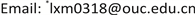Received: Nov. 27th, 2018; accepted: Dec. 12th, 2018; published: Dec. 19th, 2018ABSTRACT

In this paper, a numerical flume of internal solitary wave with various design amplitudes is established based on the Fluent software. The internal solitary wave is simulated by mass source wave-generating method and the simulation results are compared with the physical experiment and theoretical results. The data of wave-induced flow field are obtained by monitoring function of the software. Combined with the modified Morison equation, the effect of internal solitary wave on the top tension riser is calculated, and its dynamic response is analyzed by finite element method. The results indicate that the mass source wave-generating method adopted in this paper is in good agreement with the experimental and theoretical results. Under the large amplitude internal solitary wave, the horizontal force of internal solitary wave on the riser in the upper fluid is obviously greater than that on the riser in the lower fluid. The riser under the action of internal solitary wave will undergo large deformation and produce large stress. When the valley reaches the riser, the stress and deformation reach the maximum.

Keywords:Internal Solitary Wave, Numerical Simulation, Top Tensioned Riser, Dynamic Response

1中国海洋大学工程学院，山东 青岛

2山东科技大学土木工程与建筑学院，山东 青岛1. 引言

2. 造波理论与方法

2.1. 理论模型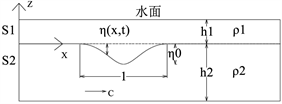Figure 1. Sketch of theoretical models for internal solitary waves

$\eta \left(x,t\right)={\eta }_{0}\mathrm{sec}{h}^{2}\left(\frac{x-ct}{l}\right)$

$\begin{array}{l}c={c}_{0}+\frac{{c}_{1}}{3}{\eta }_{0}\\ l=\sqrt{\frac{12{c}_{2}}{{c}_{1}{\eta }_{0}}}\\ {c}_{0}=\sqrt{\frac{g{h}_{1}{h}_{2}\left({\rho }_{2}-{\rho }_{1}\right)}{{\rho }_{1}{h}_{2}+{\rho }_{2}{h}_{1}}}\\ {c}_{1}=-\frac{3{c}_{0}}{2}\frac{{\rho }_{1}{h}_{2}^{2}-{\rho }_{2}{h}_{1}^{2}}{{\rho }_{1}{h}_{1}{h}_{2}^{2}+{\rho }_{2}{h}_{2}{h}_{1}^{2}}\\ {c}_{2}=\frac{{c}_{0}}{6}\frac{{\rho }_{1}{h}_{2}{h}_{1}^{2}+{\rho }_{2}{h}_{1}{h}_{2}^{2}}{{\rho }_{1}{h}_{2}+{\rho }_{2}{h}_{1}}\end{array}$

$\eta \left(x,t\right)=\frac{{\eta }_{0}}{B+\left(1-B\right){\mathrm{cosh}}^{2}\left(\frac{x-{c}_{e}t}{{l}_{e}}\right)}$

$\begin{array}{l}{c}_{e}={c}_{0}+\frac{{\eta }_{0}}{3}\left({c}_{1}+\frac{1}{2}{c}_{3}{\eta }_{0}\right)\\ {l}_{e}=\sqrt{\frac{12{c}_{2}}{\left({c}_{1}+\frac{1}{2}{c}_{3}{\eta }_{0}\right){\eta }_{0}}}\\ B=\frac{-{c}_{3}{\eta }_{0}}{2{c}_{1}+{c}_{3}{\eta }_{0}}\end{array}$

2.2. 造波方法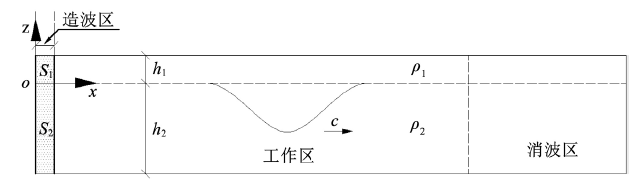Figure 2. Sketch of the mass source wave-generating method

$\begin{array}{l}\frac{\partial \rho }{\partial t}+\frac{\partial \left(\rho {u}_{i}\right)}{\partial {x}_{i}}=S\left(x,z,t\right)\\ \frac{\partial \left(\rho {u}_{i}\right)}{\partial t}+\frac{\partial \left(\rho {u}_{i}{u}_{j}\right)}{\partial {x}_{j}}=\frac{\partial }{\partial {x}_{j}}\left[\mu \frac{\partial {u}_{i}}{\partial {x}_{j}}\right]-\frac{\partial {p}_{i}}{\partial x}+\rho {g}_{i}\end{array}$

$\underset{0}{\overset{t}{\int }}{\int }_{S1}{S}_{1}\left(x,z,t\right)\text{d}{S}_{1}\text{d}t=-{\rho }_{1}\underset{0}{\overset{t}{\int }}c\eta \left(t\right)\text{d}t$

$\underset{0}{\overset{t}{\int }}{\int }_{S2}{S}_{2}\left(x,z,t\right)\text{d}{S}_{2}\text{d}t={\rho }_{2}\underset{0}{\overset{t}{\int }}c\eta \left(t\right)\text{d}t$

${S}_{1}\left(t\right)=-\frac{{\rho }_{1}c\eta \left(t\right)}{{A}_{1}}$

${S}_{2}\left(t\right)=\frac{{\rho }_{2}c\eta \left(t\right)}{{A}_{2}}$

3. 模型建立与验证

3.1. 数值水槽的建立

3.2. Fluent设置

UDF设置：利用Fluent中的DEFINE_SOURCE宏编写理论UDF，将源项添加到造波源区域，嵌入到造波区控制方程中。

3.3. 数值造波模拟结果及分析Table 1. Setting of numerical simulation condition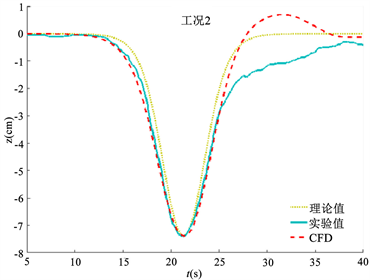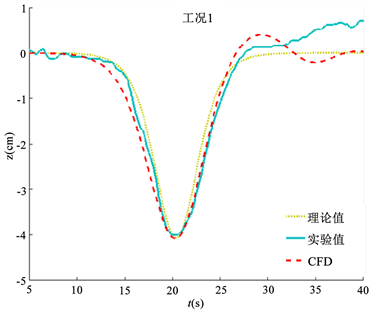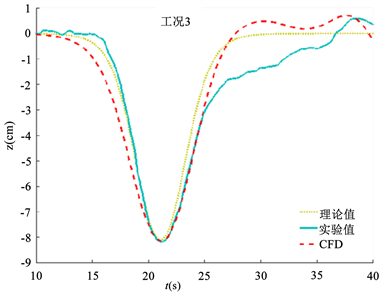Figure 3. Comparison of numerical waveform with experimental and theoretical results

4. 大振幅内波下立管的动力响应

4.1. 模型验证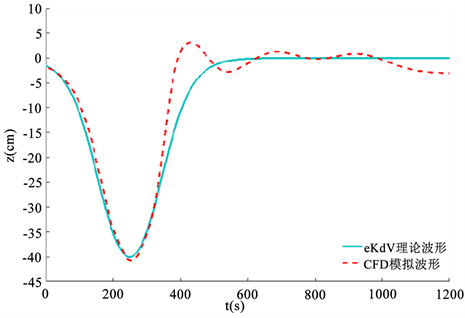Figure 4. Comparison of numerical waveform with theoretical results

4.2. 结果与分析

${f}_{x}=\frac{1}{2}{C}_{D}\rho D\left(U-\stackrel{˙}{x}\right)|U-\stackrel{˙}{x}|+{C}_{M}\rho \frac{\text{π}{D}^{2}}{4}\left(\stackrel{˙}{U}-\stackrel{¨}{x}\right)$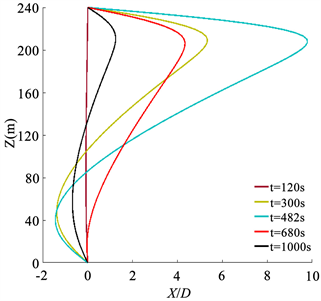Figure 5. Displacements of riser at different moments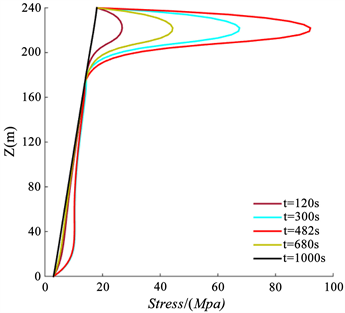Figure 6. Stresses of the riser at different moments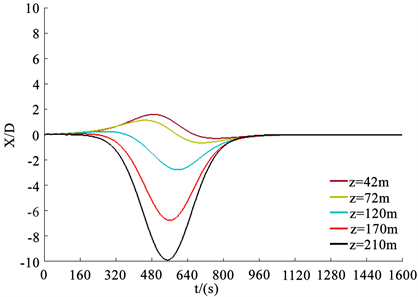Figure 7. Time-histories of displacement for nodes in different depth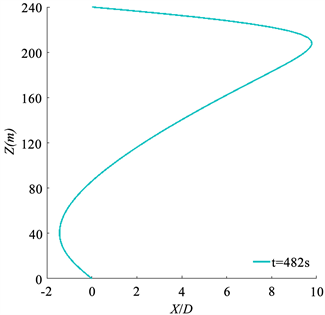Figure 8. Displacement of riser with trough arrives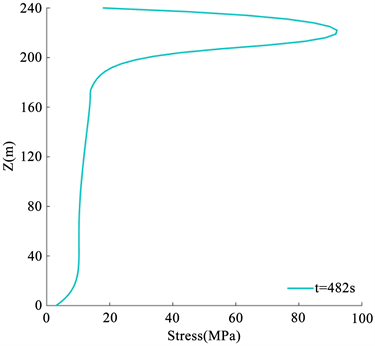Figure 9. Stresses of riser with trough arrives

5. 结论

Numerical Simulation of Internal Solitary Waves Based on Fluent and Dynamic Response Analysis of Top Tension Risers[J]. 流体动力学, 2018, 06(04): 158-168. https://doi.org/10.12677/IJFD.2018.64020

1. 1. 杜涛, 吴巍, 方欣华. 海洋内波的产生与分布[J]. 海洋科学, 2001, 25(4): 25-28.

2. 2. 高原雪, 尤云详, 王旭, 等. 基于MCC理论的内孤立波数值模拟[J]. 海洋工程, 2012, 4(14): 29-36.

3. 3. 蔡树群, 龙小敏, 甘子钧. 孤立子内波对小直径圆柱形桩柱的作用力初探[J]. 水动力学研究与进展A辑, 2002, 17(4): 497-506.

4. 4. 尤云祥, 李巍, 等. 海洋内孤立波中张力腿平台的水动力特性[J]. 上海交通大学学报, 2010, 44(1): 56-61.

5. 5. 王旭, 林忠义, 尤云祥. 两层流体中内孤立波质量源数值造波方法[J]. 上海交通大学学报, 2014, 48(6): 850-855.

6. 6. Wang, X., Lin, Z.Y., et al. (2017) Numerical Simulations for the Load Characteristics of Internal Solitary Waves on a Vertical Cylinder. Journal of Ship Mechanics, No. 9, 1071-1086.

7. 7. 黄文昊, 尤云祥, 等. 有限深两层流体中内孤立波造波实验及其理论模型[J]. 物理学报, 2013, 62(8): 1-14.

8. 8. Choi, W. and Camassa, R. (1999) Fully Nonlinear Internal Waves in a Two-Fluid Systems. Journal of Fluid Mechanics, 396, 1-36. https://doi.org/10.1017/S0022112099005820

9. 9. Helfrich, K.R. (2006) Long Nonlinear Internal Waves. Annual Review of Fluid Mechanics, 38, 395-425. https://doi.org/10.1146/annurev.fluid.38.050304.092129

10. 10. Wang, F., Wu, K.F., Guo, H.Y., et al. (2015) Experimental Study on Internal Wave Induced Flow Field. The 2nd Annual Congress Engineering and Technology (CATE), 111-116.

11. 11. 张林. 内孤立波数值造波方法及顶张力立管动力响应研究[D]: [硕士学位论文]. 青岛: 中国海洋大学, 2017.

12. 12. 高原雪. 海洋内孤立波与海洋立管的相互作用数值模拟研究[D]: [硕士学位论文]. 上海: 上海交通大学, 2012.

13. 13. Han, S.M. (2000) Non-Linear Coupled Transverse and Axial Vibration of a Compliant Structure. Part 2: Force Vibration. Journal of Sound and Vibration, 237, 875-900. https://doi.org/10.1006/jsvi.2000.3148

14. NOTES

*通讯作者。